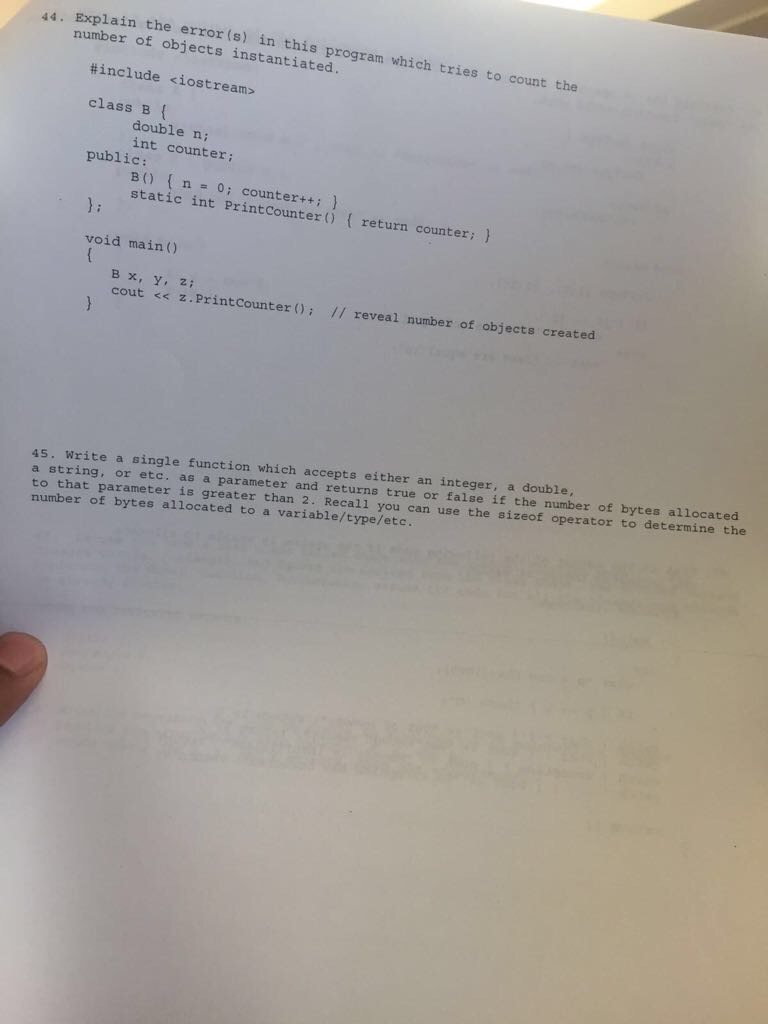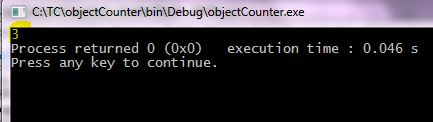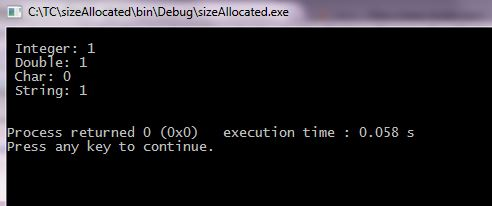# Question & Answer: Explain the error(s) in this program which tries to count the number of objects instantiated. #include class…..Explain the error(s) in this program which tries to count the number of objects instantiated. #include class B { double n: int counter: public: B () { n = 0: counter++: } static int PrintCounter() { return counter: } }: void main () { B x, y, z: cout

Don't use plagiarized sources. Get Your Custom Essay on
Question & Answer: Explain the error(s) in this program which tries to count the number of objects instantiated. #include class…..
GET AN ESSAY WRITTEN FOR YOU FROM AS LOW AS \$13/PAGE

(44)

Identifying errors:

Error 1 (in the printCounter method definition):

1.   Static functions can only access static members as they are independent of class instantiation. This line generates an error since variable counter is not a static variable

Remedy:

Mark the variable counter as static

2. Missing static object initialization

Remedy:

Initialize static variable before main function as:

int B::counter = 0;

Error 2:

1. In-built functions cout and cin exists in std namespace. Using cout generates an error as code is missing namespace declaration.

Remedy:

Include namespace as: using namespace std

Updated Program:

#include <iostream>

using namespace std;

class B {

double n;
//Mark counter as static
static int counter;

public:
B() { n = 0; counter++; }
static int PrintCounter() { return counter; }
};

//Initializing static member counter
int B::counter = 0;

int main()
{
B x, y, z;
cout << z.PrintCounter(); // reveal number of objects created
}

Sample Run:_____________________________________________________________________________________________

(45)

#include <iostream>
#include <string>

using namespace std;

//Function that accepts element and checks for number of bytes allocated
template <class T>
bool SizeAllocated(T element)
{
//Checking number of bytes allocated
if(sizeof(element) > 2)
{
return true;
}
else
{
return false;
}
}

//Main function
int main()
{
//Declaring and assigning values
int a=20;
double b=23.6;
char d=’p’;
string c=”Hello”;

//Displaying size allocated
cout << “n Integer: ” << SizeAllocated(a);
cout << “n Double: ” << SizeAllocated(b);
cout << “n Char: ” << SizeAllocated(d);
cout << “n String: ” << SizeAllocated(c);

cout << “nn”;
return 0;
}

Sample Run: